NEET  >  NCERT Based Test: Magnetic Force

# NCERT Based Test: Magnetic Force

Test Description

## 15 Questions MCQ Test Physics Class 12 | NCERT Based Test: Magnetic Force

NCERT Based Test: Magnetic Force for NEET 2023 is part of Physics Class 12 preparation. The NCERT Based Test: Magnetic Force questions and answers have been prepared according to the NEET exam syllabus.The NCERT Based Test: Magnetic Force MCQs are made for NEET 2023 Exam. Find important definitions, questions, notes, meanings, examples, exercises, MCQs and online tests for NCERT Based Test: Magnetic Force below.
Solutions of NCERT Based Test: Magnetic Force questions in English are available as part of our Physics Class 12 for NEET & NCERT Based Test: Magnetic Force solutions in Hindi for Physics Class 12 course. Download more important topics, notes, lectures and mock test series for NEET Exam by signing up for free. Attempt NCERT Based Test: Magnetic Force | 15 questions in 15 minutes | Mock test for NEET preparation | Free important questions MCQ to study Physics Class 12 for NEET Exam | Download free PDF with solutions
 1 Crore+ students have signed up on EduRev. Have you?
NCERT Based Test: Magnetic Force - Question 1

### When a magnetic compass needle is carried nearby to a straight wire carrying current, then (i) the straight wire cause a noticeable deflection in the compass needle. (ii) the alignment of the needle is tangential to an imaginary circle with straight wire as its centre and has a plane perpendicular to the wire

Detailed Solution for NCERT Based Test: Magnetic Force - Question 1

Both points are correct and these are the result of experiments done by Danish physicist Hans Christian Oersted in 1820.

NCERT Based Test: Magnetic Force - Question 2

### Which one of the following is not correct about Lorentz force?

Detailed Solution for NCERT Based Test: Magnetic Force - Question 2

If charge is not moving then the magnetic force is zero.
Since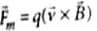As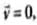for stationary charge ∴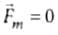.

NCERT Based Test: Magnetic Force - Question 3

### A strong magnetic field is applied on a stationary electron. Then the electron

Detailed Solution for NCERT Based Test: Magnetic Force - Question 3

As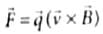As the electron is stationary. ∴ velocity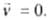∴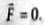So, electron will remain stationary.

NCERT Based Test: Magnetic Force - Question 4

In an inertial frame of reference, the magnetic force on a moving charged partic le is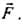Its value in another inertial frame of reference will be

Detailed Solution for NCERT Based Test: Magnetic Force - Question 4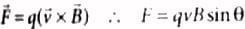which shows magnetic field is velocity dependent due to which it differs from one intertial frame to another.

NCERT Based Test: Magnetic Force - Question 5

The magnetic force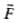on a current carrying conductor of length l in an external magnetic field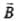is given by

NCERT Based Test: Magnetic Force - Question 6

A straight wire having mass of 1.2 kg and length of 1m carries a current of 5A. If the wire is suspended in mid-air by a uniform horizontal magnetic field, then the magnitude of field is

Detailed Solution for NCERT Based Test: Magnetic Force - Question 6

For mid-air suspension the upward force F on wire due to magnetic field B must be balanced by the force due to gravity, then
IIB = mg; B = mg/II
Here, m = 1.2 kg, g = 10 m s-2, l = 5 A, l = 1m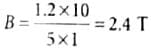NCERT Based Test: Magnetic Force - Question 7

A circular loop of radius R carrying a current I is placed in a uniform magnetic field B perpendicular to the loop. The force on the loop is

NCERT Based Test: Magnetic Force - Question 8

A 2.5 m long straight wire having mass of 500 is suspended in mid air by a uniform horizontal magnetic field B. If a current of 4 A is passing through the wire then the magnitude of the field is (Take g = 10 m s-2)

Detailed Solution for NCERT Based Test: Magnetic Force - Question 8

Here, m = 500g = 0.5kg, l = 4A, l = 2.5m
As F = IIB sinθ
mg = IIB sin90°, (∵ θ= 90°and F = mg)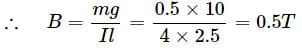NCERT Based Test: Magnetic Force - Question 9

A current of 10 A is flowing in a wire of length 1.5 m. A force of 15 N acts on it when it is placed in a uniform magnetic field of 2 T. The angle between the magnetic field and the direction of the current is

Detailed Solution for NCERT Based Test: Magnetic Force - Question 9

F = IlBsinθ or sinθ = F/IlB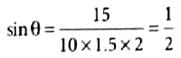or θ = 30°

NCERT Based Test: Magnetic Force - Question 10

The horizontal component of earth's magnetic field at a certain place is 3.0 x 10-5 T and having a direction from the geographic south to geographic north. The force per unit length on a very long straight conductor carrying a steady current of 1.2 A in east to west direction is

Detailed Solution for NCERT Based Test: Magnetic Force - Question 10

Force per unit length f = F/l = IB sinθ
when the current is flowing from east to west then θ = 90°, hence
f = IBsin 90° = 1.2 x 3 x 10-5 x 1 = 3.6 x 10-5 N m-1.

NCERT Based Test: Magnetic Force - Question 11

A circular coil of 20 turns and 10 cm radius is placed in a uniform magnetic field of 1.10T normal to the plane of the coil. If the current in the coil is 5A, cross-sectional area is 10-5 m2 and coil is made up of copper wire having free electron density about 1029 m-3, then the average force on each electron in the coil due to magnetic field is

Detailed Solution for NCERT Based Test: Magnetic Force - Question 11

Force on each electron,
evdB = IB/nA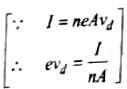Here, I = 5 A, B = 0.1 T, n = 1029 m-3
A = 10-5m2
So,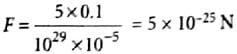NCERT Based Test: Magnetic Force - Question 12

The magnetic force per unit length on a wire carrying a current of 10 A and making an angle of 45° with the direction of a uniform magnetic field of 0.20 T is

Detailed Solution for NCERT Based Test: Magnetic Force - Question 12

l = 10 A, θ = 45°, B = 0.2 T
∴ F = IlB sin θ
∴ F/l = IB sin 45° = 10 x 0.2 x 1/√2 = 2/√2 N m-1

NCERT Based Test: Magnetic Force - Question 13

An 8 cm long wire carrying a current of 10 A is placed inside a solenoid perpendicular to its axis. If the magnetic field inside the solenoid is 0.3 T, then megnetic force on the wire is

Detailed Solution for NCERT Based Test: Magnetic Force - Question 13

F = lIB sinθ
Here, θ = 900, l = 10 A,
l = 8cm = 8 x 10-2m, B = 0.3T
F = 10 x 8 x 10-2 x 0.3 x sin 900 = 0.24 N

NCERT Based Test: Magnetic Force - Question 14

A rectangular coil ABCD is hung from one side of a balance as shown in figure. A 500 g mass is added to the other arm to balance the weight of the coil. A current of 9.8 A is passed through the coil and a constant magnetic field of 0.4 T acting inward (in xz plane) is switched on such that only arm CD of length 1.5 cm lies in the field. The additional mass m must be added to regain the balance is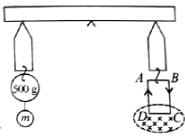Detailed Solution for NCERT Based Test: Magnetic Force - Question 14

In absence of magnetic field the weight added in one pan balances the rectangular coil in the other pan of balance,
∴ Mgl = Wcoill or Wcoil = Mg = 0.5 x 9.8 N
When current I is passed through the coil and the magnetic field is switched on.
Let m mass be added in the first pan to regain the balance
Then Mgl + mgl = Wcoil l + IBL sin90° l
mgl = IBLI
or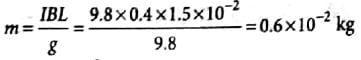= 6 x 10-3 kg = 6 g

NCERT Based Test: Magnetic Force - Question 15

Which one of the following is correct statement about magnetic forces?

Detailed Solution for NCERT Based Test: Magnetic Force - Question 15

As the action and reaction are instantaneous in IIIrd law. But magnetic forces transmit with finite speed c.

## Physics Class 12

157 videos|452 docs|213 tests
 Use Code STAYHOME200 and get INR 200 additional OFF Use Coupon Code
Information about NCERT Based Test: Magnetic Force Page
In this test you can find the Exam questions for NCERT Based Test: Magnetic Force solved & explained in the simplest way possible. Besides giving Questions and answers for NCERT Based Test: Magnetic Force, EduRev gives you an ample number of Online tests for practice

## Physics Class 12

157 videos|452 docs|213 tests

### How to Prepare for NEET

Read our guide to prepare for NEET which is created by Toppers & the best Teachers